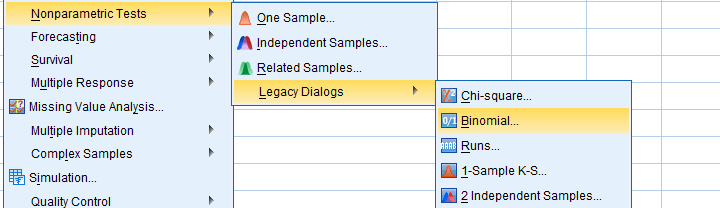SPSS TUTORIALS BASICS ANOVA REGRESSION FACTOR CORRELATION

# Overview SPSS Nonparametric Tests## Nonparametric Tests - One Sample

### SPSS Z-Test for a Single Proportion

Z-tests were introduced to SPSS version 27 in 2020.

This tutorial quickly walks you through z-tests for single proportions:

• which options should you select?
• how to interpret the output?
• which assumptions should you meet -and how to test these?
• and way more...

### Binomial Test – Simple Tutorial

A binomial test examines if a population percentage is equal to x.

Example: is 45% of all Amsterdam citizens currently single? Or is it a different percentage?

This simple tutorial quickly walks you through the basics.

### SPSS Binomial Test Tutorial

This tutorial walks you through running and interpreting a binomial test in SPSS.

### SPSS Sign Test for One Median – Simple Example

SPSS sign test for one median the right way. Recode your outcome variable into values higher and lower than the hypothesized median and test if they're distribted 50/50 with a binomial test.

## Nonparametric Tests - 2 Independent Samples

### SPSS Z-Test for Independent Proportions Tutorial

Z-tests were introduced to SPSS version 27 in 2020.

This tutorial quickly walks you through z-tests for 2 independent proportions:

• which options should you select?
• how to interpret the output?
• which assumptions should you meet -and how to test these?
• and way more...

### SPSS Mann-Whitney Test – Simple Example

The Mann-Whitney test is an alternative for the independent samples t test when the assumptions required by the latter aren't met by the data. The most common scenario is testing a non normally distributed outcome variable in a small sample (say, n < 25).

### SPSS Median Test for 2 Independent Medians

SPSS median test evaluates if two groups of respondents have equal population medians on some variable. This easy tutorial quickly walks you through.

## Nonparametric Tests - 3(+) Independent Samples

### How to Run a Kruskal-Wallis Test in SPSS?

The Kruskal-Wallis test is a nonparametric alternative for a one-way ANOVA.

This tutorial shows when to use it and how to run it in SPSS.

### SPSS Wilcoxon Signed-Ranks Test – Simple Example

SPSS Wilcoxon Signed-Ranks test is used for comparing two metric variables measured on one group of cases. It's the nonparametric alternative for a paired-samples t-test when its assumptions aren't met.

### SPSS McNemar Test

SPSS McNemar test is a procedure for testing whether the proportions of two dichotomous variables are equal. The two variables have been measured on the same cases.

### SPSS Sign Test for Two Medians – Simple Example

SPSS sign test for two related medians tests if two variables measured in one group of people have equal population medians.

### SPSS Friedman Test Tutorial

SPSS Friedman test compares the means of 3 or more variables measured on the same respondents. Like so, it is a nonparametric alternative for a repeated-measures ANOVA that's used when the latter’s assumptions aren't met.

### SPSS Cochran Q Test

SPSS Cochran's Q test is a procedure for testing whether the proportions of 3 or more dichotomous variables are equal. These outcome variables have been measured on the same people or other statistical units.

# Tell us what you think!

*Required field. Your comment will show up after approval from a moderator.

# THIS TUTORIAL HAS 7 COMMENTS:

•### By Andy on July 8th, 2020

Please give some public or environmental health related case study for binomial test.

•### By Ruben Geert van den Berg on July 8th, 2020

What about testing if the percentage of COVID infected people is equal to x? We're sure you can fill in the details from there, right?